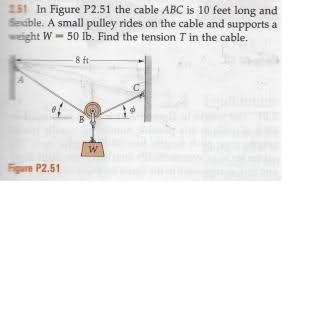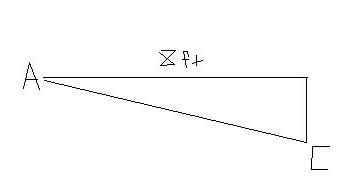# Find the tension of the system (picture included)

## Homework Statement

In order to simplify this process and avoid possible errors I have included the scanned image of the problem from my textbook. (Let me know if the picture is too small and I will upload a larger one)## Homework Equations

Mostly just free body diagrams..

## The Attempt at a Solution

For this problem I split the tensions on the right and left side of the pulley as T1(from A to B) and T2(from B to C) and once I found those out I would add them together to find the total tension of the system.
For this I know I have to find the forces in the x and y directions and I have come up with the necessary equations to solve the problem:

Fx: T2cos(phi) - T1sin(theta) (equation 1)

Fy: T2sin(phi) + T1sin(theta) - 50 (equation 2)

Using equation 1, I solved for T2 in terms of T1 and got :

T2 = T1sin(theta)/cos(phi)

Then I plugged T2 into equation 2:

T1tan(phi)sin(theta) + T1sin(theta) = 50

So my problem is basically this, I need at least one of the angles (phi or theta) for me to solve this problem, however I have very little clue as to how to obtain either one. I know I am supposed to use the fact that the length of the cable is 10feet and the distance between the walls supporting the cable is 8 feet but I really don't know how to approach this.

Thank you for any and all assistance you can provide.

PhanthomJay
Homework Helper
Gold Member

## Homework Statement

In order to simplify this process and avoid possible errors I have included the scanned image of the problem from my textbook. (Let me know if the picture is too small and I will upload a larger one)## Homework Equations

Mostly just free body diagrams..

## The Attempt at a Solution

For this problem I split the tensions on the right and left side of the pulley as T1(from A to B) and T2(from B to C) and once I found those out I would add them together to find the total tension of the system.
If the pulley is frictionless, which I assume it is, the tensions on each side are equal. In the x direction ,their x components must add algebraically to zero, and their y components must add up to the weight.
Fx: T2cos(phi) - T1sin why sin?(theta) (equation 1) equals___?

Fy: T2sin(phi) + T1sin(theta) - 50 (equation 2)equals__?

Using equation 1, I solved for T2 in terms of T1 and got :

T2 = T1sin(theta)/cos(phi)

Then I plugged T2 into equation 2:

T1tan(phi)sin(theta) + T1sin(theta) = 50

So my problem is basically this, I need at least one of the angles (phi or theta) for me to solve this problem, however I have very little clue as to how to obtain either one. I know I am supposed to use the fact that the length of the cable is 10feet and the distance between the walls supporting the cable is 8 feet but I really don't know how to approach this.

Thank you for any and all assistance you can provide.
If T1 =T2, what must the angles be?

Fx: T2cos(phi) - T1cos(theta) = 0

Fy: T2sin(phi) + T1sin(theta) = 50

I knew they had to equal 0 and 50 respectively, but the sin part was a careless error on my part.

But anyways, sp as you were saying, if t1=t2 should the angles be equal to each other?

jgens
Gold Member
I think that's what PhanthomJay is hinting at, however, I thought that would only be true if the rope was hung at equivalent heights? Perhaps a misunderstanding on my part?

That was my issue too. The fact that the cable is not at the same height led me to think that the angles were not the same. I hope I am wrong because if they do happen to be the same this would make the solution to the problem much easier.

PhanthomJay
Homework Helper
Gold Member
o sorry I missed that; so is the elevation given ?

Nope, all that is given is what is shown in the picture, the length of the cable and the distance between the 2 walls.

I know some kind of trigonometry is involved here, but I really don't know how to approach it..

At first I was considering making a triangle that goes from one wall to another so one side is 8 ft, then from point A to point C as the hypotenuse, and then from part C to the top of part like this:Would it be wrong to write the distance of the hypotenuse as 10 ft then use pythagorean theorem to determine the difference in height between A and C as 6 ft?

This is about as far as I can go..

If you use a hypotenuse of 10 ft then the sag is not taken into consideration. If the weight was fixed at a point then two tensions would exist. So, the tension in this case is expessed as

T = W/(sin(theta) + sin(phi))

The problem is to solve for sin(theta) and sin(phi). To solve, draw a vertical line through the pulley center creating two triangles. Let the horizontal distance from the left wall to the vertical line be D, then the remaining distance is 8 - D. Similarly, let the cable length from the left wall to the pulley center (the pulley is small) be L and the remaining length be 10 - L. Express the sines and cosines of theta and phi in terms of D, L, 8 - D, 10 - L. i.e.

cos(theta) = D/L, cos(phi) = (8 - D)/(10 - L), sin(theta) = sqrt(L2 - D2)/L, and
sin(phi) = sqrt((10 - L)2 - (8 - D)2)/(10 - L). Then use cos2 + sin2 =1. This will give two equations with two unknows, L and D. Solve for L and D then you can determine sin(theta) and sin(phi).

Man thanks a lot chrisk. I still have to solve for L and D but I now know exactly how to approach the problem and solve it.

thanks again